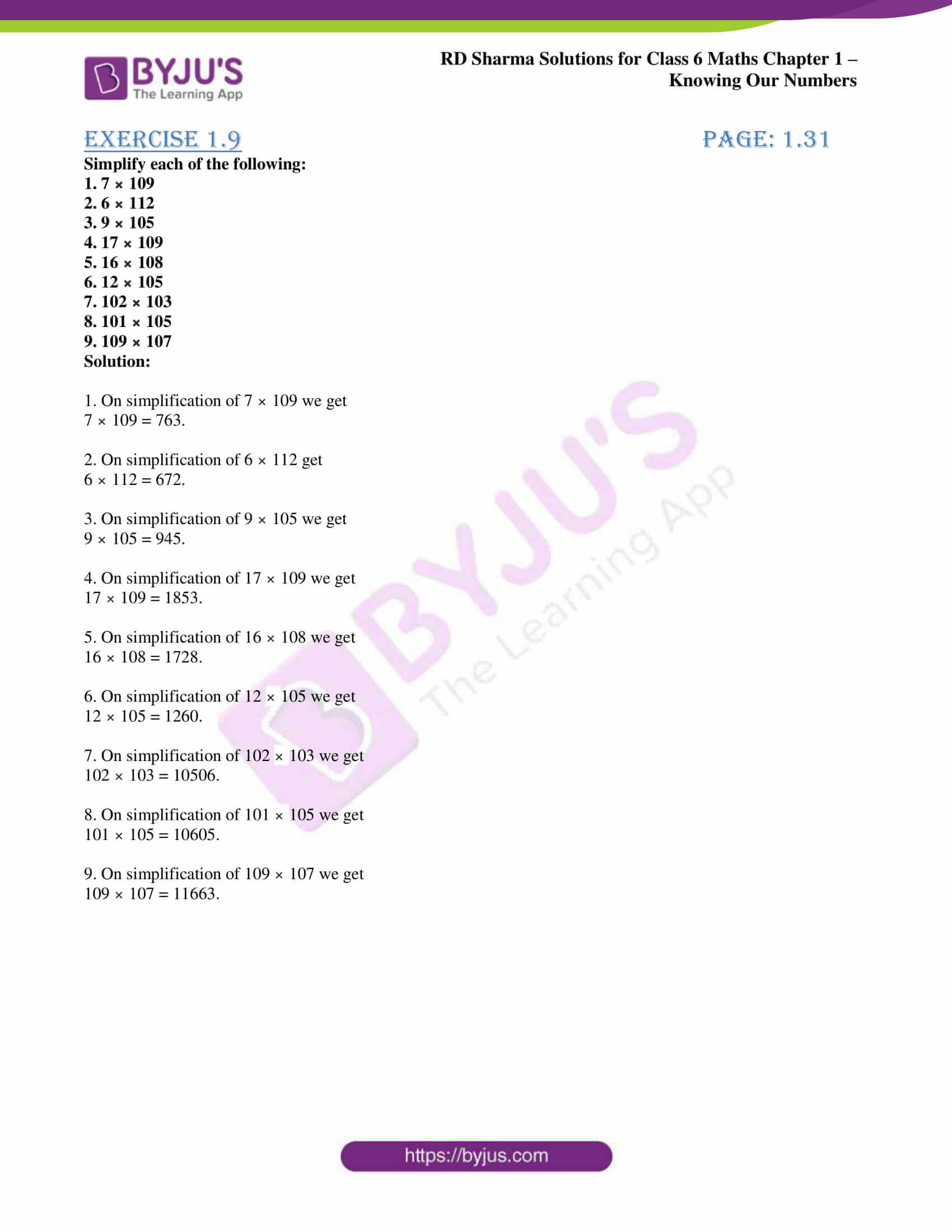# RD Sharma Solutions for Class 6 Maths Chapter 1: Knowing Our Numbers Exercise 1.9

## RD Sharma Solutions for Class 6 Maths Exercise 1.9 PDF

This exercise mainly deals with expanding of brackets while solving problems using addition, subtraction, multiplication and division. The solutions are designed by a team of subject matter experts who conduct wide research on each topic before solving problems. The main mission is to make the subject easy for students and obtain a good score in the exam. This is the ninth exercise of Chapter 1 which has a PDF of solutions that can be easily downloaded by students. RD Sharma Solutions for Class 6 Maths Chapter 1 Knowing Our Numbers Exercise 1.9 are provided here.

## RD Sharma Solutions for Class 6 Maths Chapter 1: Knowing Our Numbers Exercise 1.9 Download PDF## Access answers to Maths RD Sharma Solutions for Class 6 Chapter 1: Knowing Our Numbers Exercise 1.9

Simplify each of the following:

1. 7 × 109

2. 6 × 112

3. 9 × 105

4. 17 × 109

5. 16 × 108

6. 12 × 105

7. 102 × 103

8. 101 × 105

9. 109 × 107

Solution:

1. On simplification of 7 × 109 we get

7 × 109 = 763.

2. On simplification of 6 × 112 get

6 × 112 = 672.

3. On simplification of 9 × 105 we get

9 × 105 = 945.

4. On simplification of 17 × 109 we get

17 × 109 = 1853.

5. On simplification of 16 × 108 we get

16 × 108 = 1728.

6. On simplification of 12 × 105 we get

12 × 105 = 1260.

7. On simplification of 102 × 103 we get

102 × 103 = 10506.

8. On simplification of 101 × 105 we get

101 × 105 = 10605.

9. On simplification of 109 × 107 we get

109 × 107 = 11663.

### RD Sharma Solutions Class 6 Maths Chapter 1 – Knowing Our Numbers Exercise 1.9

RD Sharma Solutions Class 6 Maths Chapter 1 Knowing Our Numbers Exercise 1.9 outlines the methods of expanding brackets using the distributive law. The solutions are explained in a simple language to help students gain conceptual knowledge.

### Key features of RD Sharma Solutions for Class 6 Maths Chapter 1: Knowing Our Numbers Exercise 1.9

• The solutions are explained in a comprehensive manner to help students obtain overall idea about the concepts.
• The doubts which arise while solving the problems can be cleared easily with the help of solutions created by subject experts.
• The formulas and important concepts in each exercise are highlighted to make the students familiar while solving.
• The solutions are available in PDF format which can be downloaded by students for further reference.